5/5 - (1 vote)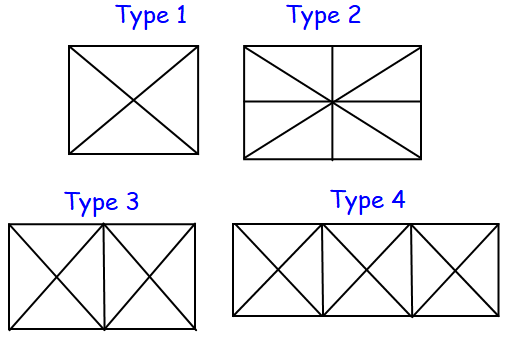Hello Friends welcome to our website GrabNaukri.com today, I am providing you Counting Figures Reasoning Tricks PDF which helps you to understand all type of ‘Counting Figures Questions’ short tricks to solve question in few seconds which asked in various competitive exams.

Details of Page Contents

## Here’s a Sneak Peek Into E-book

• Find the number of Triangles?
• Find the number of Square?
• Find the number of Quadrilateral?
• Find the number of Rectangle?

Counting Figures topic is very important for every SSC exam and you can master this topic by thorough practice. To help you with your exam preparations we have brought a free on 200+ Important Counting Figures reasoning Questions for practice.

On our website we are providing complete Notes of counting figures reasoning questions which helps you to score a good mark in the Reasoning Section in Competitive Exam by short tricks and save time in the exam for other Sections of the Exam.

In SSC Stenographer Grade C & D Exam 50 question out of 200 asked from Reasoning Section of 50 Marks. In-state Recruitment exams like DSSSB various exams, various exams, and State PSC exams, and many other exams Counting Reasoning plays a very important role to get a good score in the competitive exam.

In SSC CGL, CHSL, Tier-1 Exam 25 question of 2 marks for each question asked from Reasoning section, In SSC CPO Tier-1 Exam 50 question of 1 marks for each question asked from Reasoning Section.

This E-book contains all the Important Counting Figures reasoning Questions of Triangles, Rectangles, and Squares with short tricks. You can download the 200+ Important Counting Figures reasoning.

## Practice Questions.

Q1) Find the number of triangles in the given figure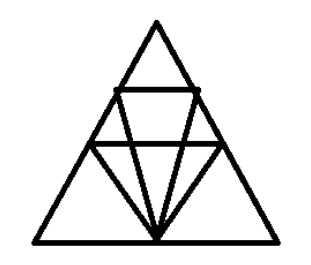(a) 12
(b) 18
(c) 22
(d) 26

Counting Figures Reasoning Tricks PDF

Q2) How many triangles are there in the given figure?(a) 20
(b) 23
(c) 24
(d) 26

Q3) How many triangles are there in the given figure?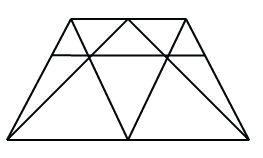(a) 18
(b) 19
(c) 20
(d) 21

Q4) How many triangles are there in the given figure?(a) 28
(b) 36
(c) 40
(d) 48

Q5) How many triangles are there in the given figure?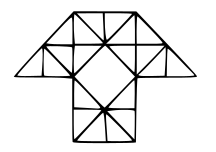(a) 29

(b) 38
(c) 40
(d) 35

People also searched for:- figure counting questions pdf, counting figures tricks, counting figures reasoning questions with answers, counting figures in reasoning tricks pdf in hindi, figure counting tricks pdf, counting figures online test, counting of figures in english, counting figures indiabix.

Important Counting Figures Questions- Free E-Book

In IBPS Banking Pre Exam one-third question asked from Reasoning Section and also in Main exam Reasoning Section has good weightage, So Reasoning is an important and scoring section for all Competitive Exams.

Q6) Find the number of triangles in the given figure.(a) 18
(b) 20
(c) 28
(d) 34

Must Read: Latest General Knowledge 2020 E-Book

Q7) Find the number of triangles in the given figure.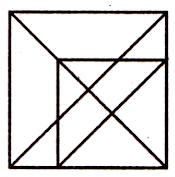(a) 16
(b) 18
(c) 20
(d) 21

Q8) Find the number of triangles and squares in the given figure.(a) 21 triangles, 7 squares

(b) 18 triangles, 8 squares
(c) 20 triangles, 8 squares
(d) 22 triangles, 7 squares